• 2021-06-01 23:57:43
import os
import numpy as np
import matplotlib.pyplot as plt

def Euclidean_distance(point1, point2): #计算两个点之间的欧几里得距离
return np.sqrt(np.sum((point1 - point2) ** 2))

def get_Euclidean_distance(point, dataSet): # 得到一个点到数据集里面所有点的距离
distanceSet = []
for sample in dataSet:
if Euclidean_distance(point, sample): # 排除这个点本身，即排除与自己距离为0的点
distanceSet.append(Euclidean_distance(point, sample))
return distanceSet

class KNN(): # k近邻算法
k = 0
def train(self, x_train, y_train): # train函数得到最好的k值，即以周围多少个点进行投票效果最好
Kmax = len(x_train) # k最大为点的个数
best_k = 0 # 初始化最好的k
best_accurrcy = 0 # 初始化最好的k对应的准确度
for k in range(1, Kmax): # 依次计算每一个k
labelSet = self.predict(x_train, k) # 计算当前k下各点的label
count = np.sum(np.logical_xor(labelSet, y_train) == 1) # 预测结果与真实标记不一致就说明预测失败
precision = 1 - count / y_train.shape # 计算在训练集上的准确度
print("k = %2d accurrcy: %.2f" % (k, precision))
if precision > best_accurrcy: # 记录最好的k
best_accurrcy = precision
best_k = k
return best_k, best_accurrcy

def predict(self, predictSet, k):
labelSet = []
for point in predictSet:
distanceSet = get_Euclidean_distance(point, x_train) # 得到当前点与训练集所有点的距离
sorted_index = sorted(range(len(distanceSet)), key=lambda k: distanceSet[k], reverse=False) # 得到距离从小到大排序的索引
count1 = list(y_train[sorted_index[:k]]).count(1) # 计算前k个最近的点的label个数，进行投票
count0 = list(y_train[sorted_index[:k]]).count(0)
if count0 < count1:
labelSet.append(1) # 哪个数字多，当前点的label就是哪个数字
else:
labelSet.append(0)
return labelSet

def plot_desicion_boundary(X, y, knn): # 画出决策边界
x_min = np.array(X)[:, 0].min() - 0.1 # 计算图的边界
x_max = np.array(X)[:, 0].max() + 0.1
y_min = np.array(X)[:, 1].min() - 0.1
y_max = np.array(X)[:, 1].max() + 0.1
xx, yy = np.meshgrid(np.arange(x_min, x_max, 0.01), np.arange(y_min, y_max, 0.01))
Z = knn.predict(np.vstack([xx.ravel(), yy.ravel()]).T.tolist(), knn.k)
Z = np.array(Z).reshape(xx.shape)
f, axarr = plt.subplots(1, 1, figsize=(10, 8))
axarr.contourf(xx, yy, Z, alpha = 0.4)
axarr.scatter(np.array(X)[:, 0], np.array(X)[:, 1], c=y, s=10, edgecolor='k')
axarr.set_title("KNN (k={})".format(1))
plt.savefig("k={}.png".format(1))
plt.show()

path = os.path.abspath(os.path.dirname(__file__)) # 获取py文件当前路径
x_train = np.loadtxt(path + "/30alpha.csv", delimiter=",", usecols=(0, 1))
y_train = np.loadtxt(path + "/30alpha.csv", delimiter=",", usecols=(2))

knn = KNN() # 实例化KNN模型
best_k, best_accurrcy = knn.train(x_train, y_train) # 得到最好的k
print("best k =", best_k, " best accurrcy:", best_accurrcy)
knn.k = best_k # 记录最好的k
plot_desicion_boundary(x_train, y_train, knn) # 画出决策边界


与python文件同目录下的西瓜3.0数据集文件30alpha.csv

0.697,0.46,1
0.774,0.376,1
0.634,0.264,1
0.608,0.318,1
0.556,0.215,1
0.403,0.237,1
0.481,0.149,1
0.437,0.211,1
0.666,0.091,0
0.243,0.267,0
0.245,0.057,0
0.343,0.099,0
0.639,0.161,0
0.657,0.198,0
0.36,0.37,0
0.593,0.042,0
0.719,0.103,0


输出：

k = 1 accurrcy: 0.94
k = 2 accurrcy: 0.88
k = 3 accurrcy: 0.82
k = 4 accurrcy: 0.65
k = 5 accurrcy: 0.82
k = 6 accurrcy: 0.76
k = 7 accurrcy: 0.76
k = 8 accurrcy: 0.76
k = 9 accurrcy: 0.65
k = 10 accurrcy: 0.53
k = 11 accurrcy: 0.47
k = 12 accurrcy: 0.47
k = 13 accurrcy: 0.47
k = 14 accurrcy: 0.53
k = 15 accurrcy: 0.53
k = 16 accurrcy: 0.53
best k = 1 best accurrcy: 0.9411764705882353

决策边界：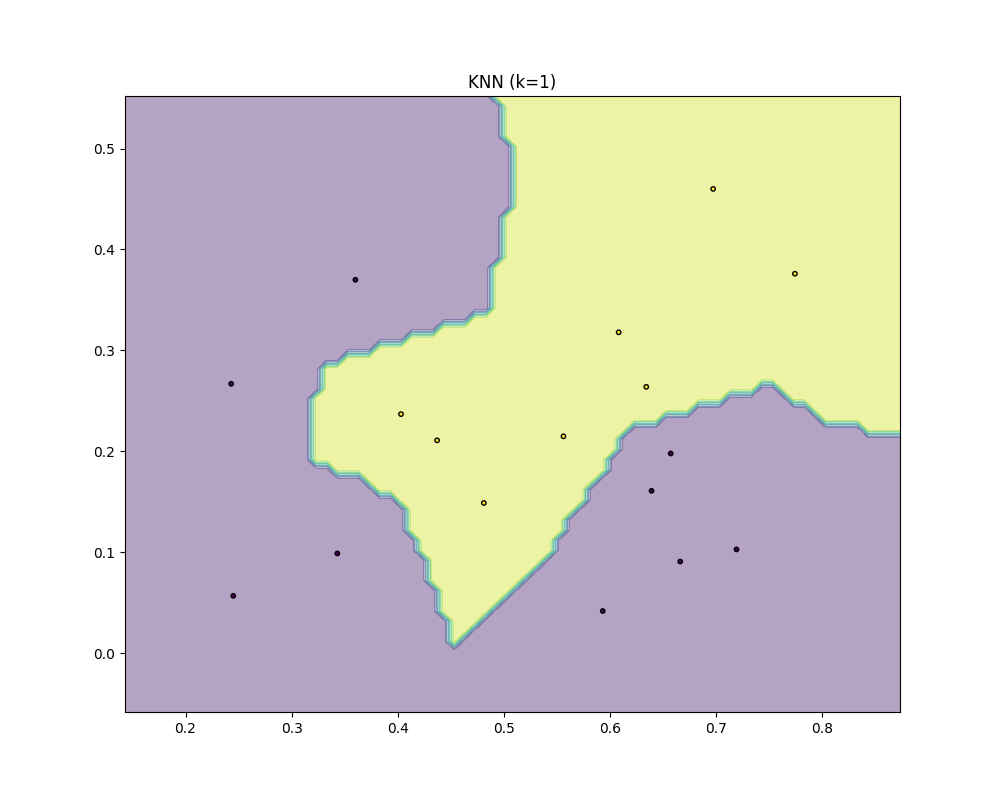References

决策边界用python实现 - 路途陌客 - 博客园

画出使用分类器得到的决策边界_dengjiaxing0321的博客-CSDN博客

Python实现画出使用分类器得到的决策边界_Cassie_pyLu的博客-CSDN博客

numpy中的ravel()、flatten()、squeeze()的用法与区别_tymatlab的专栏-CSDN博客_.ravel()

Numpy中stack()，hstack()，vstack()函数详解_张康的博客-CSDN博客_vstack

python matplotlib contour画等高线图_Mr_Cat123的wudl博客-CSDN博客

ljpzzz/machinelearning

西瓜书《机器学习》课后答案–chapter10 _10.1 KNN算法_CodeTutor-CSDN博客

han1057578619/MachineLearning_Zhouzhihua_ProblemSets机器学习 西瓜书
更多相关内容
• 此代码将找出二维数据集的决策边界。 文件包含多个支持函数，主程序是 DecisionBoundary_SVMs.m 示例集包含线性和非线性数据集，使用带有 RGF 核的 SVM，我们将找出数据集的决策边界matlab
• 获取数据集，并画图代码如下： import numpy as np from sklearn.datasets import make_moons import matplotlib.pyplot as plt # 手动生成一个随机的平面点分布，并画...# 咱们先顶一个一个函数来画决策边界 def plo
• 在这里，选择两个类来获得两个类之间的最佳决策边界。 这些类是二维（双变量）和一维（单变量）。 它被称为二分类器。 分类器本身在三种情况下被简化： 情况 1：- 在这种情况下，特征向量在统计上是独立的，...matlab
• 该代码实现了libsvm-3.23工具箱中SVDD的决策边界可视化，其实现流程为： 1. 建立训练集的SVDD超球体模型 2. 利用网格法填充训练集区域 3. 预测每个网格点的得分 4. 根据网格点得分绘制等高线 5. 绘制决策边界 ...
• 1）KNN算法基础知识： KNN全称K Nearest Neighbor, k是指最近邻居的个数。 俗话说物以类聚，人以群分，我们通常判别一个人是好是坏的方式就是看他周围是一群好人还是坏人。 这个算法也是如此，假如A的周围有一堆好人...
• 使用pandas和numpy实现的knn，包括了基于matplotlib的数据可视化，决策边界可视化等，喜欢研究机器学习原理的小伙伴们来下载哟～～～ 使用jupyter-notebook或者jupyter-lab玩耍哟～～～
• H = viewboundary（TREE）返回图形的句柄包含决策边界的可视化分类树 TREE。 H = viewboundary(TREE,'PARAM1',VALUE1,'PARAM2',VALUE2,...) 指定可选参数名称-值对： “Beta”标量常数用于照亮决策边界区域与数据点...matlab
• 而散点图更是包含着属于不同类别的数据点（用颜色或形状表示），决策边界可以通过多种不同的策略绘制：单线决策边界和基于轮廓的决策边界，这一节主要以逻辑回归为例分析如何绘制线性和非线性决策边界

决策边界的重要性/意义
在使用数据集训练机器学习模型之后，我们通常需要可视化特征空间中数据点的类。散点图上的决策边界就是出于这个目的。而散点图更是包含着属于不同类别的数据点（用颜色或形状表示），决策边界可以通过多种不同的策略绘制：

单线决策边界：在散点图上绘制决策边界的基本策略是找到一条将数据点分隔成不同类区域的单线。现在，利用训练过的模型找到与机器学习算法相关的参数，进而找到这条直线。然后利用得到的参数和机器学习算法找到直线坐标。如果你不知道ML算法的工作原理，那么你将无法继续进行下去。

基于轮廓的决策边界：另一种策略是绘制轮廓，这些轮廓是用匹配或紧密匹配的颜色包围数据点的区域——描绘数据点所属的类，以及描绘预测类的轮廓。这是最常用的策略，因为它不使用模型训练后得到的机器学习算法的参数和相关计算。但另一方面，我们并不能很好地用一条直线来分离数据点，也就是说这条直线只能通过训练后得到的参数及其坐标计算得到。

### 1 决策边界

在二分类问题中，我们只需要一个线性判别函数 𝑓 ( 𝒙 ; 𝒘 ) = 𝒘 T 𝒙 + 𝑏 𝑓(𝒙; 𝒘) = 𝒘^T𝒙 + 𝑏 。特征空间 R 𝐷 \mathbb{R}^𝐷 中所有满足 𝑓 ( 𝒙 ; 𝒘 ) = 0 𝑓(𝒙; 𝒘) = 0 的点组成一个分割超平面（Hyperplane），称为决策边界（Decision Boundary）或决策平面（Decision Surface）。决策边界将特征空间一分为二，划分成两个区域，每个区域对应一个类别。

超平面是纯粹的数学概念，不是物理概念，它是平面中的直线、空间中的平面的推广，只有当维度大于3，才称为“超”平面。通常，在1维空间里超平面为数轴上的一个点，在2维空间中的超平面是一条线，在3维空间中的超平面是一个平面。一个超平面可以将它所在的空间分为两半, 它的法向量指向的那一半对应的一面是它的正面, 另一面则是它的反面。

决策边界主要分为线性决策边界（linear decision boundaries）和非线性决策边界（non-linear decision boundaries）。注意：决策边界是假设函数的属性，由参数决定，而不是由数据集的特征决定。下面主要举一些例子，形象化的来说明线性决策边界和非线性决策边界。

对于下面的数据分布，图中这条直线可以比较完美地将数据分成两类。如下：如果我们遇到下图的情况，我们就不能用一个直线将其进行分类了，而是可以用一个圆将数据进行分类。下面来看一下非线性的决策边界的例子：### 2 逻辑回归的线性决策边界

#### 2.1 原理简介

对于逻辑回归原理的详细介绍，可以阅读：线性分类（三）-- 逻辑回归 LR

回顾逻辑回归分类的原理：通过训练的方式求出一个 n + 1 n+1 维权值向量 w \pmb{w} ，每当新来一个样本 x i \pmb{x}_i 时，与参数 w \pmb{w} 进行点乘，结果带入sigmoid函数，得到的值为该样本发生（即 y ^ = 1 \hat y =1 事件发生）的概率值。如果概率大于0.5，分类为1；否则分类为0。
对于下面的公式：
p ^ = σ ( z ) = 1 1 + e ( − z ) z = w T ⋅ x i (1) \hat p = \sigma(z) = \frac {1} {1 + e^{(-z)}} \qquad z = \pmb{w}^T \centerdot \pmb{x}_i \tag{1}
当 z > 0 z > 0 时， 1 < 1 + e ( − z ) < 2 1 < 1 + e^{(-z)} < 2 ，因此 p ^ > 0.5 \hat p > 0.5 ; 当 z < 0 z < 0 时， 2 < 1 + e ( − z ) 2 < 1 + e^{(-z)} ，因此 p ^ < 0.5 \hat p < 0.5 ;

也就是，其中有一个边界点 w T ⋅ x i = 0 \pmb{w}^T \centerdot \pmb{x}_i=0 ，大于这个边界点，分类为1，小于这个边界点。我们称之为决策边界（decision boundary）。

决策边界： w T ⋅ x i = 0 \pmb{w}^T \centerdot \pmb{x}_i=0 同时也代表一个直线： 假设 X X 有两个特征 x 1 、 x 2 x_1、x_2 ，那么有 w T ⋅ x i = w 0 + w 1 x 1 + w 2 x 2 = 0 \pmb{w}^T \centerdot \pmb{x}_i= w_0 + w_1x_1 +w_2x_2=0

这是一个直线，可以将数据集分成两类。可以改写成我们熟悉的形式：
x 2 = − w 0 − w 1 x 1 w 2 (2) x_2 = \frac {-w_0 - w_1x_1} {w_2} \tag{2}

#### 2.2 具体实现

使用鸢尾花数据集，画出决策边界。首先可视化数据集
1. 导入相关的包

import numpy as np
import matplotlib.pyplot as plt
from sklearn import datasets
from sklearn.model_selection import train_test_split
from sklearn.linear_model import LogisticRegression
from sklearn.metrics import accuracy_score


2. 导入数据集

iris = datasets.load_iris()
X = iris.data
y = iris.target
X = X[y<2, :2]
y = y[y<2]
plt.scatter(X[y==0,0], X[y==0,1], s=10, c='b', marker='o')
plt.scatter(X[y==1,0], X[y==1,1], s=10, c='r', marker='x')
plt.xlabel('sepal_length')
plt.ylabel('sepal_width')
plt.show()3. 训练模型

X_train, X_test, y_train, y_test = train_test_split(X, y)
model_logistic_regression = LogisticRegression()
model_logistic_regression.fit(X_train, y_train)
y_predict = model_logistic_regression.predict(X_test)
print(accuracy_score(y_test, y_predict))
print(model_logistic_regression.coef_)
print(model_logistic_regression.intercept_)


4. 绘制决策边界

# 生成一条直线，x为4~8范围内的1000点 y为x2函数的调用
coef = np.array(model_logistic_regression.coef_)
x1_plot = np.arange(4, 8, step=0.1)
x2_plot = -(x1_plot * coef + model_logistic_regression.intercept_)/coef
plt.plot(x1_plot, x2_plot)
plt.scatter(X[y==0,0], X[y==0,1], s=10, c='b', marker='o')
plt.scatter(X[y==1,0], X[y==1,1], s=10, c='r', marker='x')
plt.xlabel('sepal_length')
plt.ylabel('sepal_width')
plt.title('Decision Boundaray')
plt.show()可以发现，对于逻辑回归算法来说，其得到的决策边界就是一条直线，所以本身还是线性回归算法中的一种，其分类标准本质就是点落在线的上面还是下面，按此分为两类。

不过对于多项式来说，就不能简单的求出一条直线的方程了，这样就需要绘制一个不规则的决策边界的方法，在平面中，分布着无数个点，对于每一个点，都使用模型来判断一下其分为哪一类，最后绘制出来的颜色块就是决策边界。

5. 图像绘制函数

简单叙述就是，将X轴和Y轴分为数个点，相应的都使用每个进行预测，对应分配一些颜色属性

# 绘制决策边界的方法
from matplotlib.colors import ListedColormap
def plot_decision_boundary(model, axis):

x0,x1 = np.meshgrid(
np.linspace(axis,axis,int((axis-axis)*100)).reshape(-1,1),
np.linspace(axis,axis,int((axis-axis)*100)).reshape(-1,1)
)
X_new = np.c_[x0.ravel(),x1.ravel()]
y_predict = model.predict(X_new)
zz = y_predict.reshape(x0.shape)
custom_cmap = ListedColormap(['#EF9A9A', '#FFF59D', '#90CAF9'])

plt.contourf(x0, x1, zz, linewidth=5, cmap=custom_cmap)

plot_decision_boundary(model_logistic_regression, axis=[4,7.5,1.5,4.5])
plt.scatter(X[y==0,0], X[y==0,1], s=10, c='b', marker='o')
plt.scatter(X[y==1,0], X[y==1,1], s=10, c='r', marker='x')
plt.show()### 3 逻辑回归的非线性决策边界

#### 3.1 多项式回归

其核心思想就是换元法，比如圆的表达式： x 1 2 + x 2 2 − r 2 = 0 x_1^2+x_2^2-r^2=0 ，将上式中的 x 1 2 x_1^2 整体看作第一个特征 m 1 m_1 ，将 x 2 2 x_2^2 整体看作第二个特征 m 2 m_2 ，那么则可以转换成线性回归的问题：其中 m 1 m_1 前的系数为1， m 1 m_1 前系数也为1，且 r 2 r^2 是系数 θ 0 \theta_0

这样就从 m 1 、 m 2 m_1、m_2 来说还是线性边界，针对于 x 1 、 x 2 x_1、x_2 来说变成了非线性的圆形决策边界。这就从线性回归转换成多项式回归，同理为逻辑回归添加多项式项，就可以对非线性的方式进行比较好的分类，决策边界就是曲线的形状。
相当于为样本多添加了几个特征，这些特征是原先样本的多项式项（像是 X 2 X^2 就是对 X X 进行了平方），增加了这些特征以后就可以使用线性回归的思路，来更好的拟合原来的数据，本质上就是，求出了原来的特征而言的非线性的曲线，即为了更好地拟合数据进行了升维

需要注意的是，思路方面，对数据集的操作里，PCA是对数据集进行降维处理，而多项式回归是让数据集进行升维，在升维以后可以更好地拟合高维的数据，这两种要看情况使用。

#### 3.2 代码实现

加载相关包，然后设置一个随机数，种子为666，生成 X X y y X X 为两百个样本，每个样本为两个特征，是第一个特征的平方和第二个特征的平方相加小于1.5，小于1.5为1，大于为0，然后绘制图像。

1. 导入相关包

import numpy as np
import matplotlib.pyplot as plt
np.random.seed(666)

X = np.random.normal(0, 1, size=(200, 2))
y = np.array((X[:,0]**2+X[:,1]**2)<1.5, dtype='int')
plt.scatter(X[y==0,0], X[y==0,1])
plt.scatter(X[y==1,0], X[y==1,1])
plt.xlabel('First Feature')
plt.ylabel('Second Feature')
plt.show()2. 特征映射

def feature_mapping(x1, x2, degree, as_ndarray=False):
"""
特征映射
"""
data = {"f{}{}".format(i - p, p): np.power(x1, i - p) * np.power(x2, p)
for i in np.arange(degree + 1)
for p in np.arange(i + 1)
}
if as_ndarray:
return np.array(pd.DataFrame(data))
else:
return pd.DataFrame(data)


3. Sigmoid函数

def sigmoid(z):
"""
Sigmoid Function
"""
return(1/(1+np.exp(-z)))


4. 损失函数
Logistic Regression正则化代价函数为：
J ( θ ) = 1 m ∑ i = 1 m [ − y i log ⁡ ( h θ ( x i ) ) − ( 1 − y i ) log ⁡ ( 1 − h θ ( x i ) ) ] + λ 2 m ∑ j = 1 n θ j 2 (3) J\left( \theta \right)=\frac{1}{m}\sum\limits_{i=1}^{m}{[-{{y}_{i}}\log \left( {{h}_{\theta }}\left( {{x}_{i}} \right) \right)-\left( 1-{{y}_{i}} \right)\log \left( 1-{{h}_{\theta }}\left( {{x}_{i}} \right) \right)]}+\frac{\lambda }{2m}\sum\limits_{j=1}^{n}{\theta _{j}^{2}}\tag{3}

def regularized_cost(theta, X, y, learning_rate=0.1):
"""
加入正则化
"""
theta_j1_to_n = theta[1:]
regularized_term = (1/(2*len(X))) * np.power(theta_j1_to_n, 2).sum()
total_cost = np.mean(-y*np.log(sigmoid(X @ theta)) - (1-y)*np.log(1-sigmoid(X @ theta))) + regularized_term


5. 梯度下降
正则化梯度为：
∂ J ( θ ) ∂ θ j = ( 1 m ∑ i = 1 m ( h θ ( x i ) − y i ) ) + λ m θ j  for j ≥ 1 (4) \frac{\partial J\left( \theta \right)}{\partial {{\theta }_{j}}}=\left( \frac{1}{m}\sum\limits_{i=1}^{m}{\left( {{h}_{\theta }}\left( {{x}_{i}} \right)-{{y}_{i}} \right)} \right)+\frac{\lambda }{m}{{\theta }_{j}}\text{ }\text{for j}\ge \text{1} \tag{4}

def regularized_gradient(theta, X, y, lam=1):
'''
加入正则化
'''
theta_j1_to_n = theta[1:]
regularized_theta = (lam / len(X)) * theta_j1_to_n
regularized_term = np.concatenate([np.array(), regularized_theta])
total_gradient = (1 / len(X)) * X.T @ (sigmoid(X @ theta) - y) + regularized_term



6. 预测

def predict(x, theta):
prob = sigmoid(x @ theta)
return (prob >= 0.5).astype(int)


7. 拟合参数

data = feature_mapping(X[:,0], X[:,1], degree=6)
theta = np.zeros(data.shape)

res = opt.minimize(fun=regularized_cost, x0=theta, args=(data, y), method='Newton-CG', jac=regularized_gradient)


8. 决策边界

def draw_boundary(power, lam):
#     """
#     power: polynomial power for mapped feature
#     lam: lambda constant
#     """
density = 1000
threshhold = 2 * 10**-3

final_theta = feature_mapped_logistic_regression(power, lam)
x1, x2 = find_decision_boundary(density, power, final_theta, threshhold)
plt.scatter(X[y==0,0], X[y==0,1])
plt.scatter(X[y==1,0], X[y==1,1])
plt.xlabel('First Feature')
plt.ylabel('Second Feature')

plt.scatter(x1, x2, c='y', s=10, marker='x')
plt.title('Decision boundary')
plt.show()

def feature_mapped_logistic_regression(power, lam):
#     """for drawing purpose only.. not a well generealize logistic regression
#     power: int
#         raise x1, x2 to polynomial power
#     lam: int
#         lambda constant for regularization term
#     """

data = feature_mapping(X[:,0], X[:,1], power, as_ndarray=True)
theta = np.zeros(data.shape)

res = opt.minimize(fun=regularized_cost,
x0=theta,
args=(data, y, lam),
method='TNC',
final_theta = res.x

return final_theta

def find_decision_boundary(density, power, theta, threshhold):
"""
寻找决策边界函数
"""
t1 = np.linspace(-4, 4, density)
t2 = np.linspace(-4, 4, density)

cordinates = [(x, y) for x in t1 for y in t2]
x_cord, y_cord = zip(*cordinates)
mapped_cord = feature_mapping(x_cord, y_cord, power)  # this is a dataframe

inner_product = np.array(mapped_cord) @ theta

decision = mapped_cord[np.abs(inner_product) < threshhold]

return decision.f10, decision.f01

draw_boundary(power=6, lam=100)#lambda=1#### 3.3 管道

看了一些博客，发现可以通过pipeline（管道）实现。首先引用pipeline这个类，进行实例化，对pipeline进行构造，其中的列表就是对应的每一个步骤的对应的类，第一步进行多项式的过程，第二步进行数据归一化，第三步就是进行线性回归，这就创建了一个管道。然后引用PolynomialFeatures类，使用方法同样的，先进行实例化，传入参数degree，其表示为原来的数据集添加的最高的幂，这里设置为2，这就初始化好了，最后fit一下 X X 。详细过程参考：PolynomialFeatures的运算逻辑

from sklearn.linear_model import LinearRegression
from sklearn.preprocessing import PolynomialFeatures
from sklearn.pipeline import Pipeline
from sklearn.preprocessing import StandardScaler

# 为逻辑回归添加多项式项的管道
def PolynomialLogisticRegression(degree):
return Pipeline([
('poly', PolynomialFeatures(degree=degree)),
('std_scaler', StandardScaler()),
('log_reg', LogisticRegression())
])

# 使用管道得到对象
poly_log_reg = PolynomialLogisticRegression(degree=2)
poly_log_reg.fit(X, y)

print(poly_log_reg.score(X, y))
"""
输出：0.96
"""
plot_decision_boundary(poly_log_reg, axis=[-4, 4, -4, 4])
plt.scatter(X[y==0,0], X[y==0,1])
plt.scatter(X[y==1,0], X[y==1,1])
plt.show()当degree较大时，会发生过拟合现象，此时可以引入一个新参数C，和惩罚项penalty。计算方式为： C ⋅ J ( θ ) + L 2 C\cdot J(\theta) + L_2 ，如果C越大，在优化损失函数的时候就可以更好更快的将前项减到最小，这种就是在sklearn中的使用的方式。

def PolynomialLogisticRegression(degree,C=0.1,penalty='l2'):
return Pipeline([
('poly',PolynomialFeatures(degree=degree)),
('std_scaler',StandardScaler()),
('log_reg',LogisticRegression(C=C,penalty=penalty))
])



补充：

在现实生活中和一些高级项目中，都会涉及到许多特征。那么，如何在二维散点图中绘制决策边界呢？

面对这种情况，通常有多种解决办法：

1. 利用随机森林分类器等给特征进行重要性评分，得到2个最重要的特征，然后在散点图上绘制决策边界。

2. 主成分分析（PCA）或线性判别分析（LDA）等降维技术可用于将N个特征嵌入到2个特征中，从而将N个特征的信息解释或减少为2个特征（n_components = 2）。然后再基于这两个特征在散点图上绘制决策边界。

### 参考

展开全文多项式回归 pipeline
• 逻辑回归是一种针对不同类别数据的分类方法，以预测数据点属于一个类别还是另一个类别。 Sigmoid 假设函数用于计算 y 属于特定类别的概率。 使用 Zscore 对训练数据进行标准化。matlab
• 逻辑回归案例二：鸢尾花数据分类，决策边界绘制逐步代码讲解1 数据加载2 数据EDA3 模型创建及应用3.1 数据切分3.2 创建模型与分类3.3 决策边界绘制3.3.1 二分类决策边界绘制3.3.2 多分类决策边界绘制3.3.3 三维决策...

### 逻辑回归案例二：鸢尾花数据分类，决策边界绘制逐步代码讲解

手动反爬虫，禁止转载： 原博地址 https://blog.csdn.net/lys_828/article/details/121929869（CSDN博主：Be_melting）

 知识梳理不易，请尊重劳动成果，文章仅发布在CSDN网站上，在其他网站看到该博文均属于未经作者授权的恶意爬取信息


# 1 数据加载

导入模块和加载数据

import numpy as np
import pandas as pd
import seaborn as sns
import matplotlib.pyplot as plt
sns.set(color_codes=True)
%matplotlib inline

import warnings
warnings.filterwarnings('ignore')



输出结果如下。（也可以直接从sklearn中导入dataset数据集，里面包含了鸢尾花数据）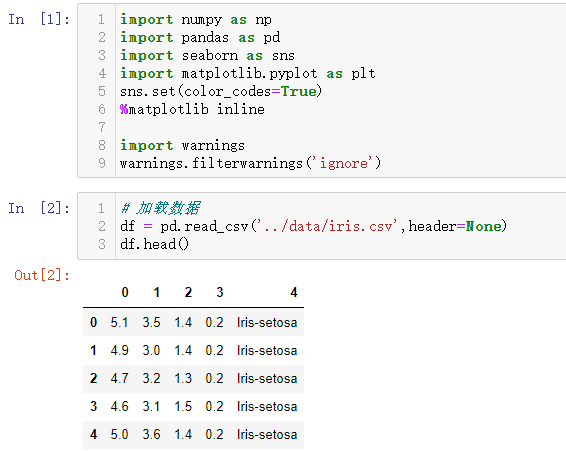关于iris数据集中的介绍，其中前四个字段就是花萼和花瓣的长和宽，加载图片说明如下。

from IPython.display import Image
Image("../data/iris1.png")
Image("../data/iris2.png")


输出结果如下。iris数据集一共150条数据，共包含了3类花，各50条数据。对应的四个字段的名称图中也有给出。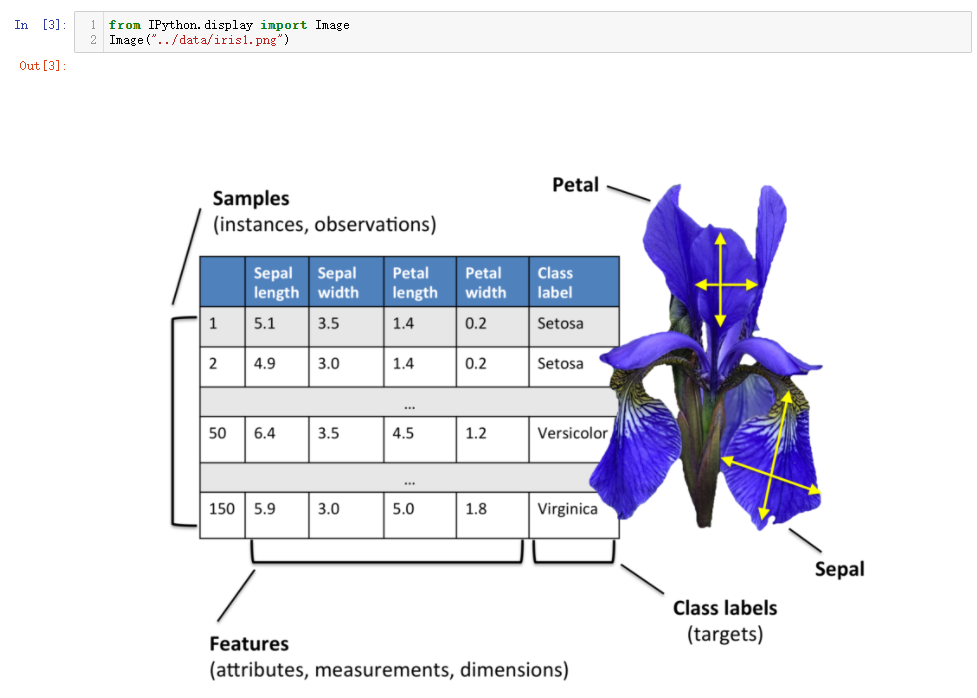对应的三种花的样式，下图中也可以做个直观展示。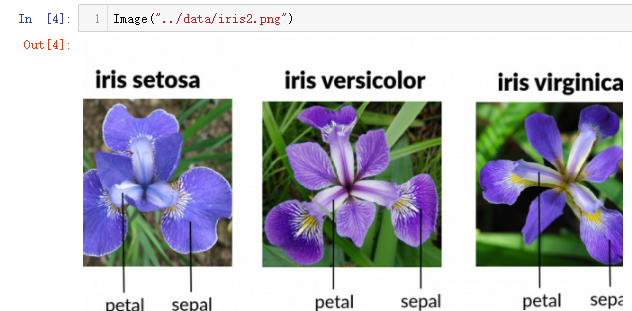将字段名称修改后指定为读入的数据集，代码如下。

col_name =   ['sepal_length', 'sepal_width', 'petal_length', 'petal_width', 'species']
df.columns = col_name


输出结果如下。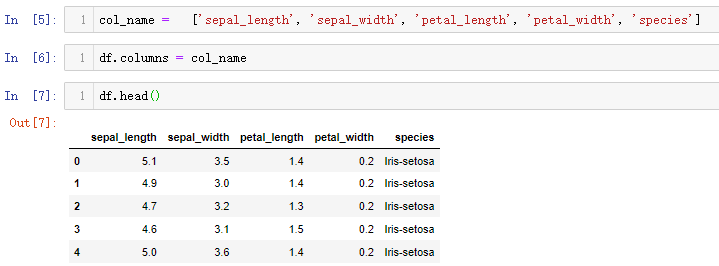# 2 数据EDA

数据读取后进行探索式分析，首先为了保证原始数据的完整性，尽量在进行分析之前进行数据备份，然后任意选取两个字段进行散点图绘制，查看相关关系。

iris = df.copy()

plt.figure(figsize=(8,8))
sns.scatterplot(data=iris,x='petal_length' ,y='petal_width')


输出结果如下。比如查看花瓣(petal)的长度和宽度之间的关系。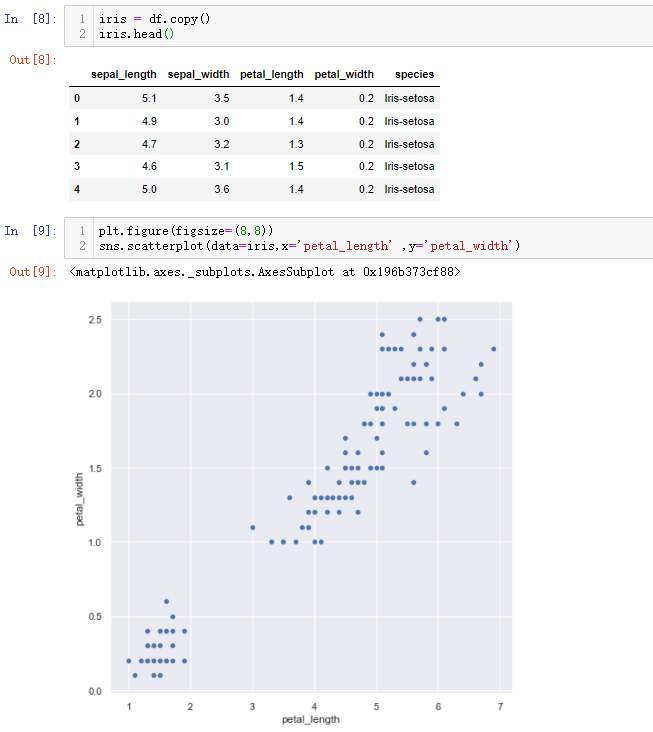可以借助hue参数，实现分类数据显示，代码如下。

iris.species.unique()
plt.figure(figsize=(5,5))
sns.scatterplot(data=iris,x='petal_length' ,y='petal_width' ,hue='species')


输出结果如下。可以看出不同种类的花瓣的长和宽是有一个较为明显的区分。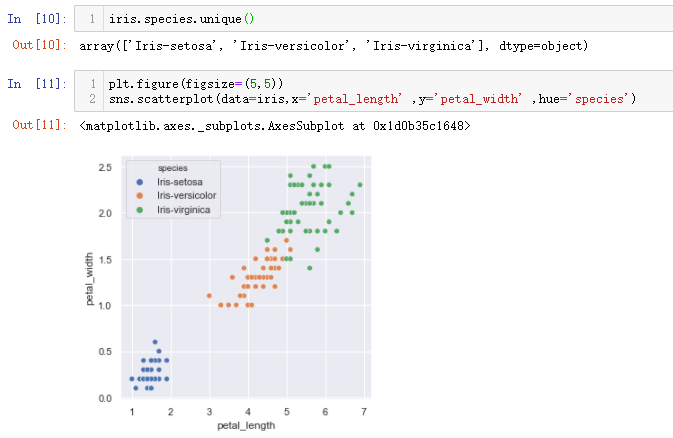上面两个字段只是随机选择，可能凑巧数据分类的散点图层次较为清晰，更好的方式展现四个字段的关系还是需要使用pairplot绘制，代码如下。

# 通过统计函数 绘制数据点位，核实各种类的数量
print(iris.groupby('species').size())
sns.pairplot(iris,hue='species',size=1.8)


输出结果如下。通过中间对角线的分布图，可以看到每两个字段对应的三种花之间的关联，三条曲线重叠的范围越多，说明彼此之间交叉混合的数据越多，凭借着这两个字段来分别三种花的难度越大。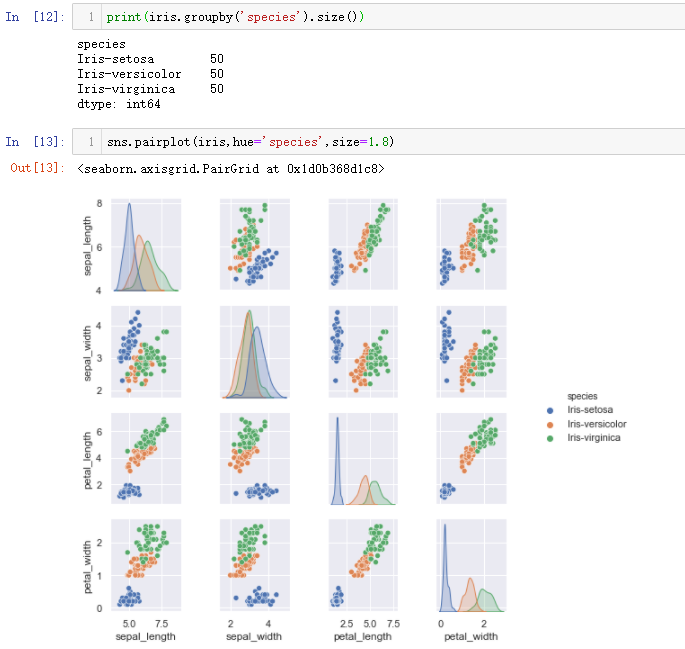比如单独在探究一下花萼(sepal)长宽与三种花分类之间的关联，绘制分类散点图代码如下。

plt.figure(figsize=(7,7))
sns.scatterplot(data=iris,x='sepal_length' ,y='sepal_width' ,hue='species')


输出结果如下。可以清晰分辨出iris-setosa和剩下两类花之间的关系，但是剩下的两类花之间的数据彼此交叉，无法直接将其分类清晰，所以如果选择这两个字段进行分类依据最后得到的模型效果也不会太好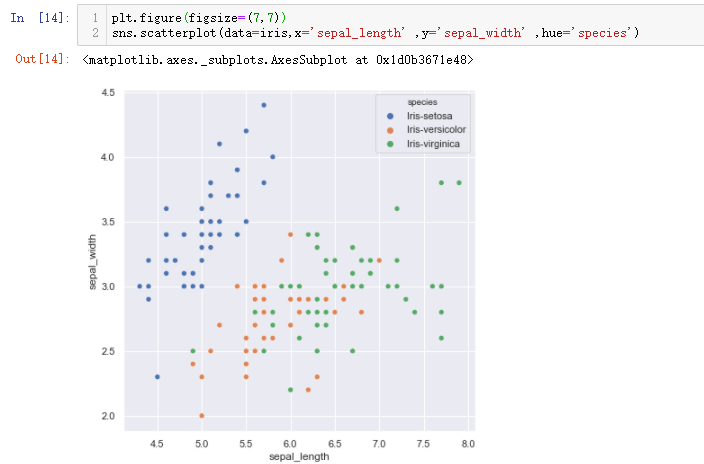# 3 模型创建及应用

直接通过EDA可以发现，需要通过绘制paiplot图形然后进一步通过人为的选择对应的字段进行三种花的分类，肉眼可能会存在着一定的误差，而直接采用模型来帮忙就能解决这个问题，把所有的字段都给扔进模型，让模型自己学习，最后得到一个最佳结果。

## 3.1 数据切分

首先要把数据准备好，先处理特征字段和标签字段，代码如下。

features = iris.iloc[:, :-1].values
features.shape
labels = iris.iloc[:, -1].values
labels.shape


输出结果如下。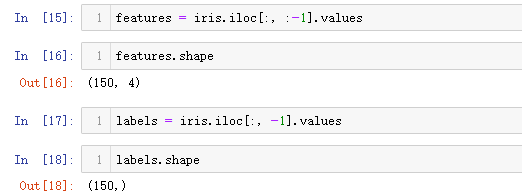接着就是划分训练集和测试集数据，划分比例为8:2，代码如下。

from sklearn.model_selection import train_test_split
X_train, X_test, y_train, y_test = train_test_split(features,labels, test_size=0.2)
X_train.shape
X_test.shape


输出结果如下。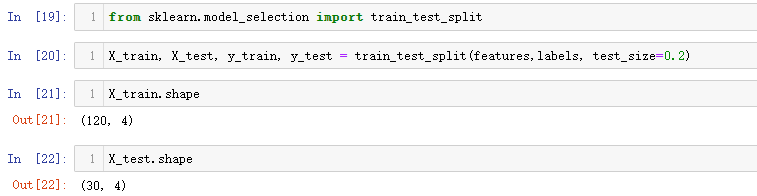## 3.2 创建模型与分类

逻辑回归的模型创建和应用的过程要比线性回归多一步，就是进行结果分类，前面的步骤都是一致，代码操作如下。

#第一步：导入模型函数
from sklearn.linear_model import LogisticRegression
#第二步：进行模型初始化
model = LogisticRegression()
#第三步：模型训练
model.fit(X_train,y_train)
#第四步：模型得分/评估
model.score(X_test,y_test)
#第五步：模型预测
model.predict(X_test)


输出结果如下。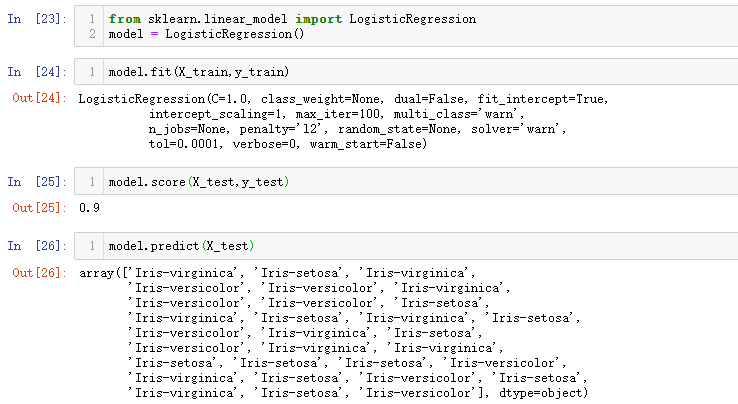以上步骤和线性回归模型分析过程一模一样，至于逻辑回归区别就在于最后的分类上面，需要利用预测的结果，借助混淆矩阵进行可视化展示，代码如下。

y_predicted = model.predict(X_test)
model.intercept_ ,model.coef_

from sklearn.metrics import confusion_matrix
cm = confusion_matrix(y_test,y_predicted)
cm
plt.figure(figsize = (10,7))
sn.heatmap(cm, annot=True)
plt.xlabel('Predicted')
plt.ylabel('Truth')


输出代码如下。逻辑回归是基于线性回归基础上，也可以输出截距和斜率。混淆矩阵的使用很简单，只需要把对应的模块导入后把测试数据和预测数据传入，就自动得到分类的结果，通过热力图可以更加直观地展示。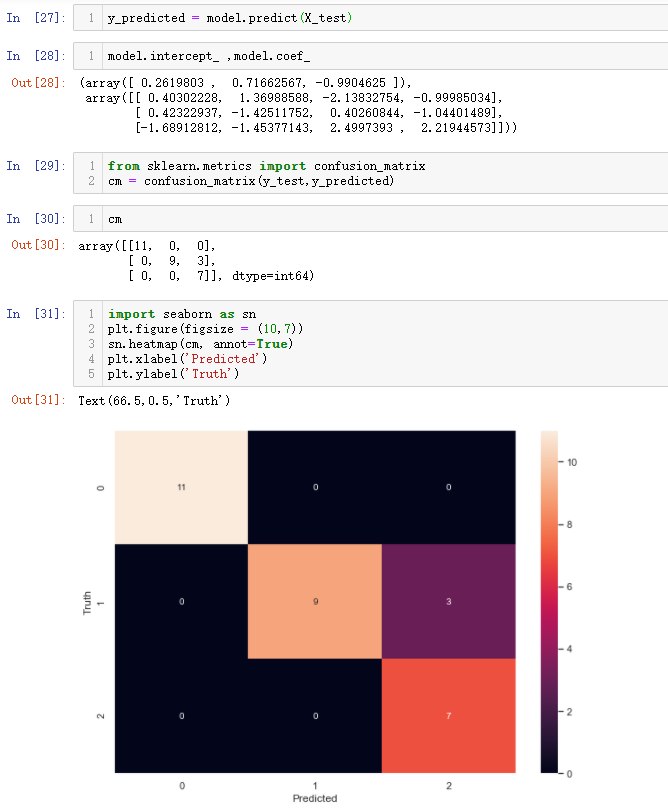结果解读需要借助一下计数器，由于没有提前进行标签数据的数值化处理，热力图上出来的值并不知道如何对应三种花的信息，因此可以通过预测结果和真实结果的计数比对来确定，代码如下。

from collections import Counter
Counter(list(y_test))

Counter(list(y_predicted))


输出结果如下。热力图中编号为0的是Iris-setosa类别，而热力图中的3个错误分类表示模型把编号1错误分类为编号2，结合着第二行和第三列各自的总和，可以知道编号1是为Iris-versicolor，编号2是Iris-cirginica。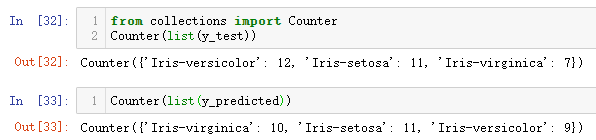## 3.3 决策边界绘制

通过上面的一阵分析，可以发现，如果不对标签字段进行字符串编码，最后的输出结果要经过一阵子分析才能知道对应的结果，所以对于字段数据在传入模型时，尽量进行编码处理，比如将三种花的类型进行编码，探究具体数据是如何进行分类。

### 3.3.1 二分类决策边界绘制

首先查看一下数据，核实标签数据中的分类名称。

iris
iris.species.unique()
sns.scatterplot(data=iris, x="sepal_length", y="sepal_width",hue='species')


输出结果如下。根据之前按照花萼长和宽绘制的散点图，可以发现三种类别的分类较为复杂，所以为了搞清楚模型是怎么进行分类？可以先取两类数据进行测试。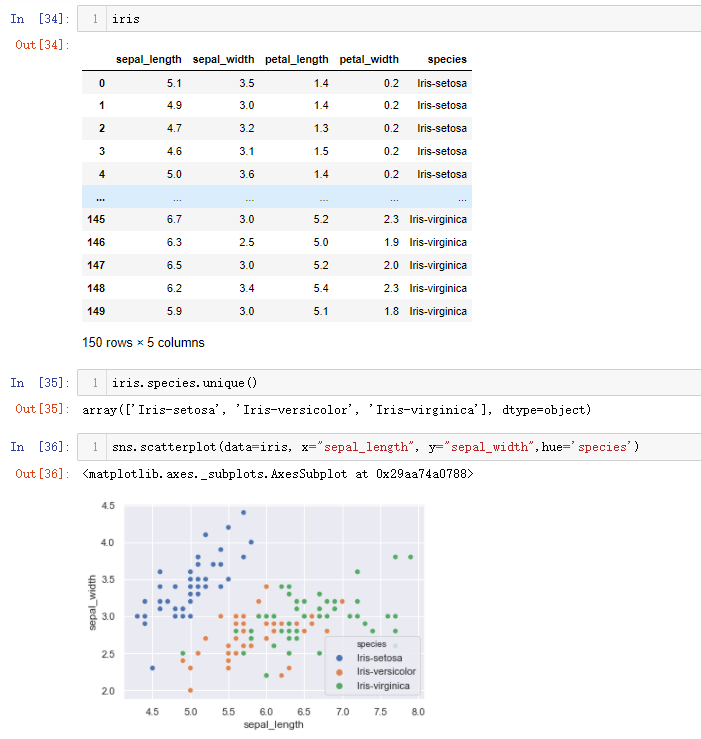但是需要把标签字段的编码工作完成，代码操作如下。

def func1(t):
if t == 'Iris-setosa' :
return int(0)
elif t == 'Iris-versicolor':
return int(1)
elif t =='Iris-virginica':
return int(2)

iris2 = iris.copy()
iris2['species'] = iris2['species'].apply(func1)


输出结果如下。为了防止原始数据被破坏，还是重新进行备份，然后在进行操作。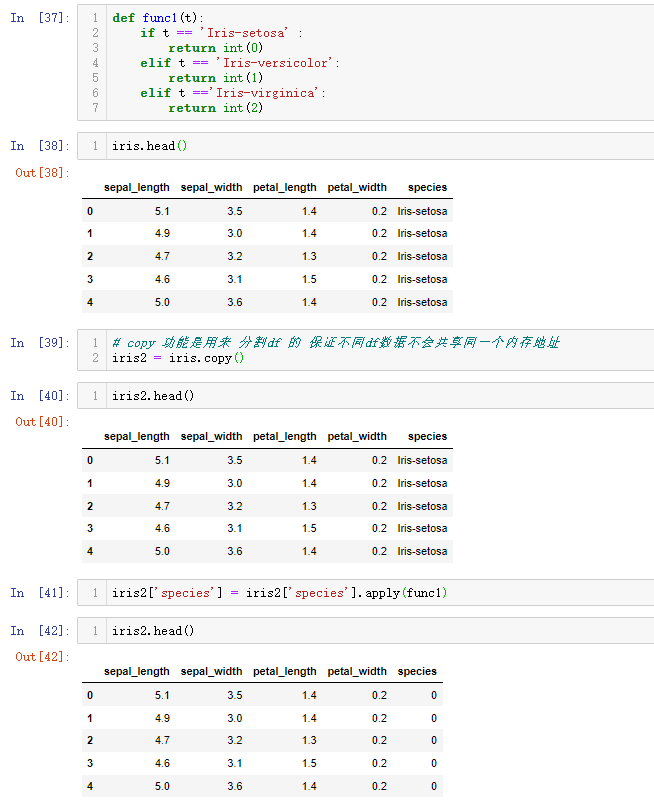再次核实下标签字段中的分类名称，并进行某一分类信息的剔除，比如删除第三类数据，代码操作如下。

iris2['species'].unique()
iris2 = iris2[iris2.species != 2]
iris2['species'].unique()
iris2.shape


输出结果如下。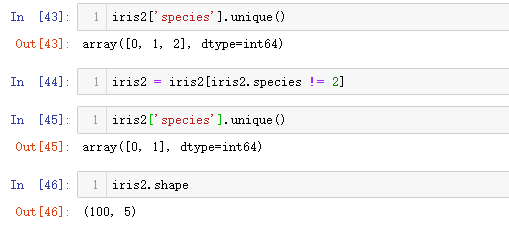然后对剩下两类花进行散点图绘制，查看具体的数据分布情况，代码如下。

sns.scatterplot(data=iris2, x="sepal_length", y="sepal_width",hue='species')


输出结果如下。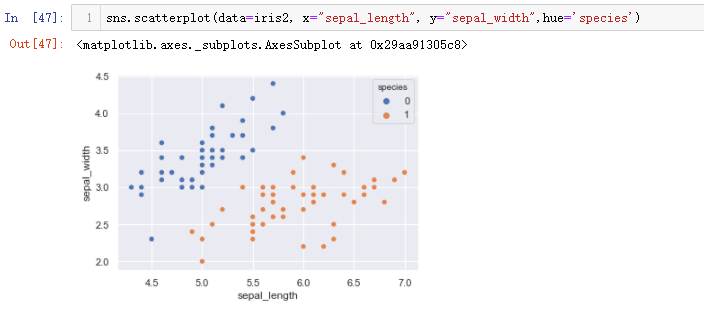上图中的分类结果是直接利用seaborn中封装的函数进行，接下来第一步要做的就是利用模型来进行分类。还是要执行3.1和3.2中的流程，代码如下。

#数据切分
features2 = iris2.iloc[:, :2].values
labels2 = iris2.iloc[:, -1].values
#模型初始化
model2 = LogisticRegression()
#模型训练
model2.fit(features2,labels2)
#模型评估
model2.score(features2,labels2)


输出结果如下。通过人眼可以直接进行分类的数据，通过模型进行分类，最后的得分也可以高达99%。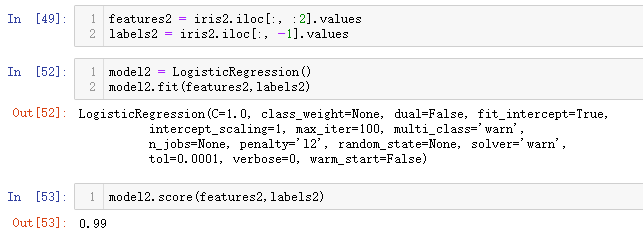最后就剩下最重要的问题，既然可以实现99%分类正确率，如何进行决策边界的绘制？首先需要获取标签字段中的最小和最大值，代码如下。

features2[:5]
x_min, x_max = features2[:, 0].min() - 0.5, features2[:, 0].max() + 0.5
y_min, y_max = features2[:, 1].min() - 0.5, features2[:, 1].max() + 0.5
x_min, x_max
y_min, y_max


输出结果如下。这里加减0.5是为了后面绘制画布扩大点距离。通过绘制分类散点图核实一下大致区间无误，同时也可以对比没有进行分类的数据图形，代码及输出如下。图形界面上x和y轴的上下限是在上方索取的范围之间，核实无误。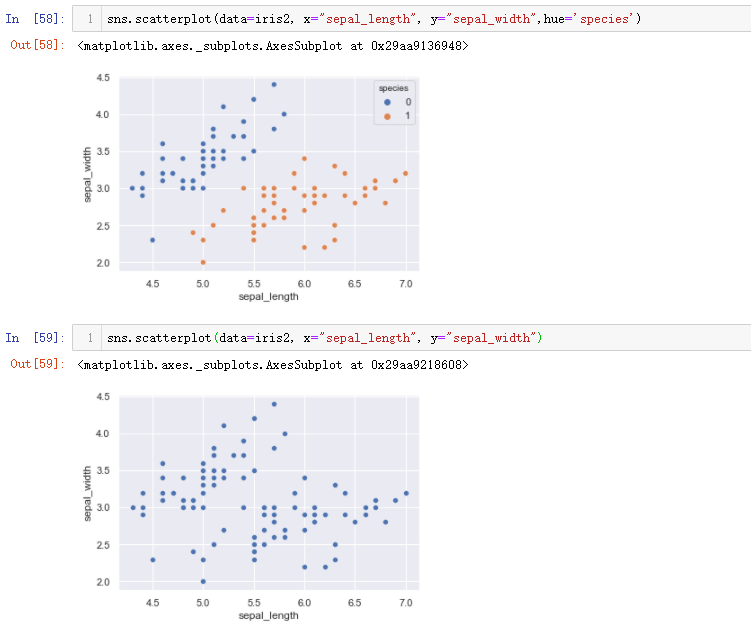模型训练好了之后是要进行使用，而绘制决策边界的思想就是在指定的x和y轴指定范围围成的区域内生成很多数据点，然后用模型预测这些数据点，就会形成一个分类的区分，再对两边的数据的进行着色处理就可以得到决策边界。

假定按照0.02的距离生成数据点，布满整个区域，可以利用arange()方法，然后结合这meshgrid()就完成了类似棋盘的绘制。可以想象一下五子棋盘的样式，这里构建的数据就是棋盘上的每一个交点的坐标数据，代码如下。

h=0.02
xx, yy = np.meshgrid(np.arange(x_min, x_max, h), np.arange(y_min, y_max, h))
xx.shape
yy.shape


输出结果如下。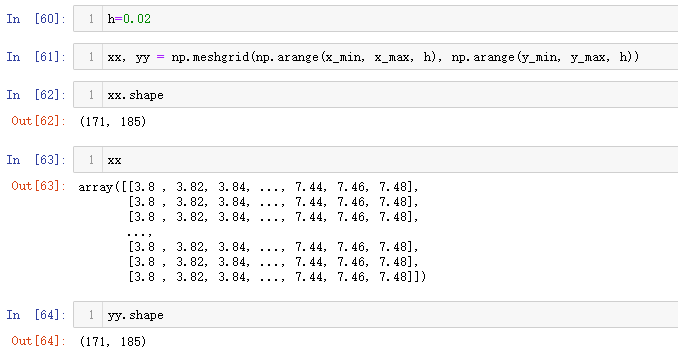棋盘绘制完毕后，利用训练好的模型对棋盘中的每一个交点进行预测。但是需要注意，模型预测时候传递的数据类型是二维的数据结构，因此需要把数据给拍扁，结合之前numpy中介绍的ravel()方法就可以实现，代码如下。

xx.ravel()
Z = model2.predict(np.c_[xx.ravel(), yy.ravel()])
Z


输出结果如下。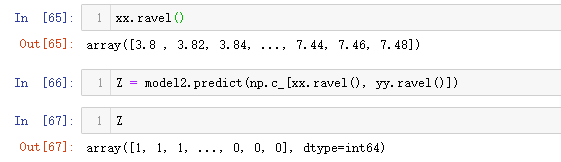自此模型就把网盘上的交点都预测出对应的结果，剩下的就是进行绘制图形，具体需要使用到pcolormesh()方法，测试使用代码如下。

Z = Z.reshape(xx.shape)
plt.figure(1, figsize=(6, 6))
plt.pcolormesh(xx, yy, Z, cmap=plt.cm.Paired)


输出结果如下。这里有一个留意的地方，预测出来的结果是一个一维数据结果，要在图形x和y轴上显示就必须转化为原来的数据形状大小，直接进行reshape()即可。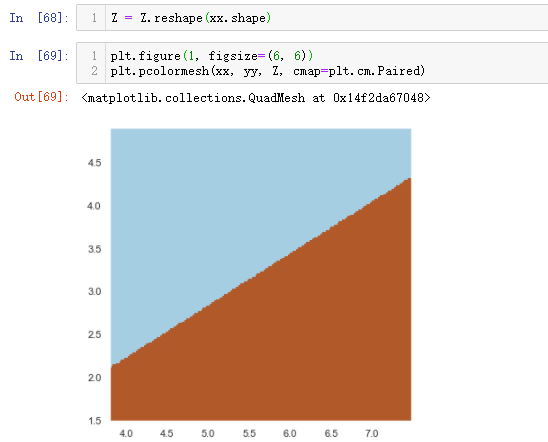使用pcolormesh()方法绘制图像就和使用散点图绘制的方式类似，都是把对应的数据传入，不过这里是根据x和y轴的信息，将预测的z值绘制出来。再添加上二分类的数据，代码如下。

plt.figure(1, figsize=(6, 6))
plt.pcolormesh(xx, yy, Z, cmap=plt.cm.Paired)
plt.scatter(features2[:, 0], features2[:, 1], c=labels2, edgecolors="k", cmap=plt.cm.Paired)
plt.xlabel("Sepal length")
plt.ylabel("Sepal width")

plt.xlim(xx.min(), xx.max())
plt.ylim(yy.min(), yy.max())
plt.xticks(())
plt.yticks(())


输出结果如下。图中分割线处锯齿形状就是说明这个图形是由一个个点组成。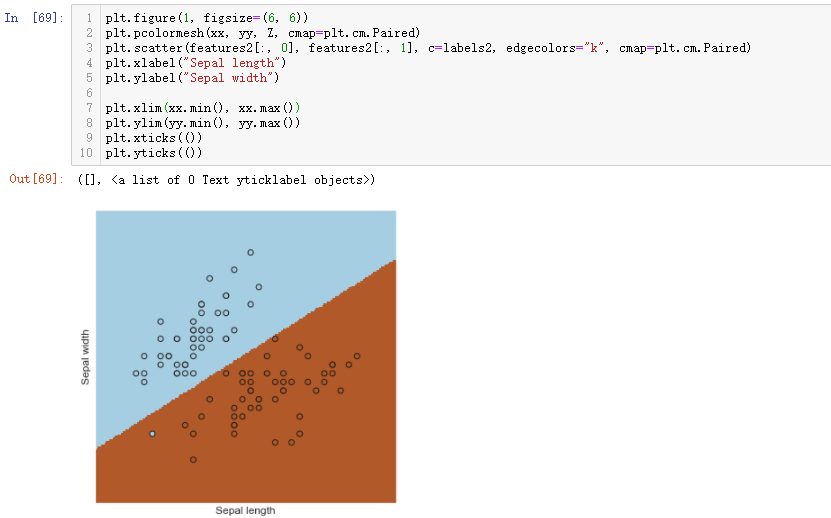对比一下使用seaborn中hue参数进行绘制的分类图，代码及输出结果如下。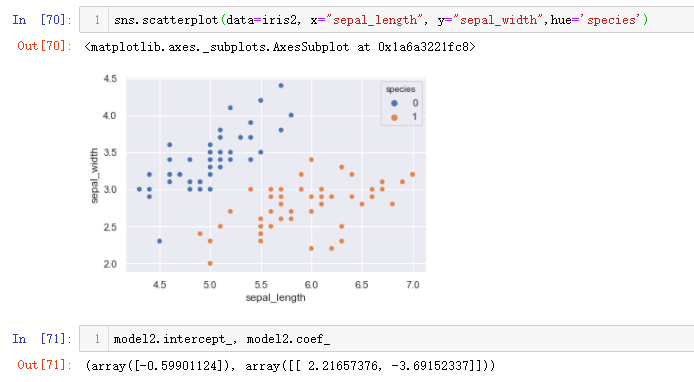然后也可以输出最终决策边界的截距和斜率，至此二分类决策边界的绘制就介绍完毕。

### 3.3.2 多分类决策边界绘制

依旧重新备份一下数据，将标签数据进行编码处理，然后再通过seaborn中的分类散点图快速查看数据分布，代码如下。

iris3 = iris.copy()
iris3['species']=iris3['species'].apply(func1)
sns.scatterplot(data=iris3, x="sepal_length", y="sepal_width",hue='species')


输出结果如下。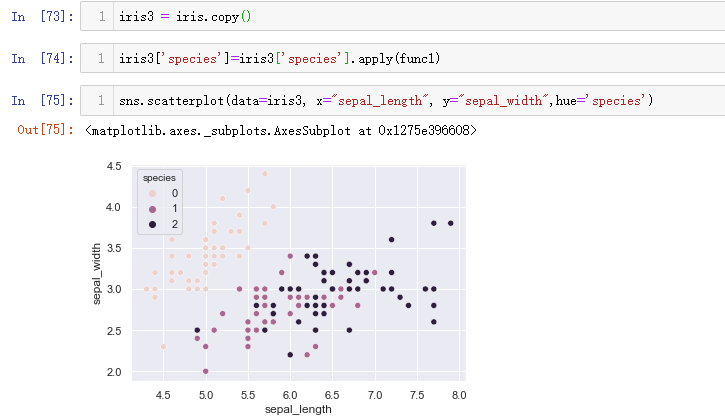接着进行数据集切分和模型创建及预测的操作，代码如下。

iris3.head()
features3 = iris3.iloc[:, :2].values
labels3 = iris3.iloc[:, -1].values

model3 = LogisticRegression()
model3.fit(features3,labels3)
model3.score(features3,labels3)


输出结果如下。此次模型的分类预测得分明显降了下来，只达到了76.7%。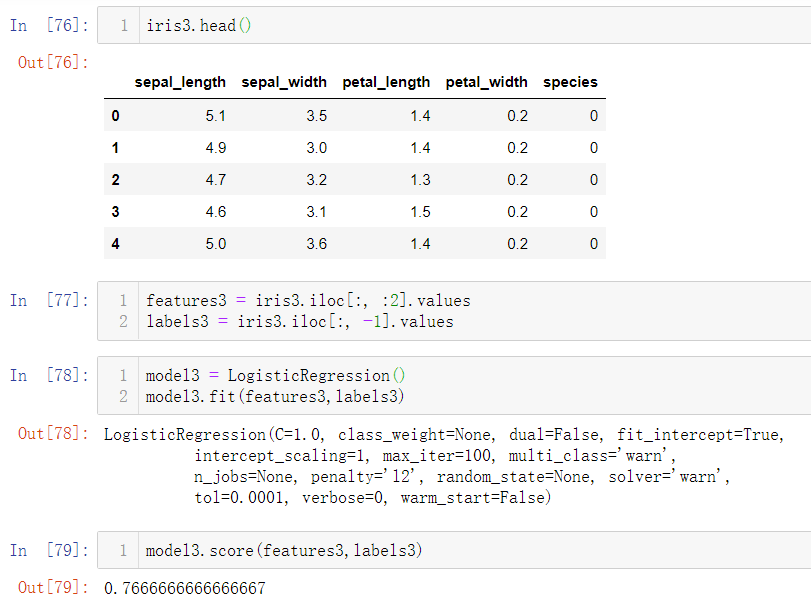通过混淆矩阵来看一下分类的结果，代码如下。

y_predicted = model3.predict(features3)

from sklearn.metrics import confusion_matrix
cm = confusion_matrix(labels3,y_predicted)

plt.figure(figsize = (10,7))
sn.heatmap(cm, annot=True)
plt.xlabel('Predicted')
plt.ylabel('Truth')


输出结果如下。这里的过程和之前的一致，最主要的就是进行了标签字段数据的编码这里的0,1,2可以一一对应上，不需要再进行计数分析了。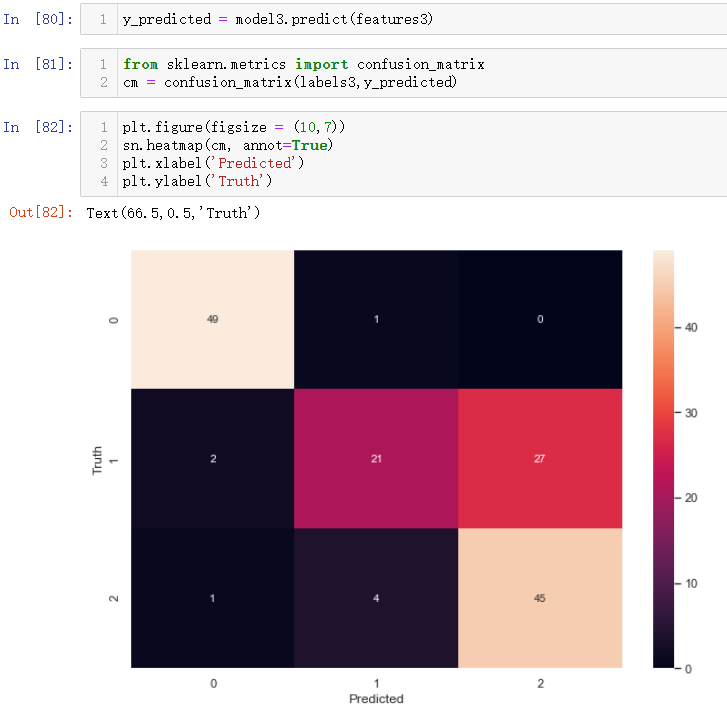在可视化的热图上也可以清晰看出编号1类花和编号2类的花之间确实难以进行划分，按照二分类决策边界绘制的思路，应用到当前数据中，流程一模一样，代码如下。

#指定x和y轴取值范围
x_min, x_max = features3[:, 0].min() - 0.5, features3[:, 0].max() + 0.5
y_min, y_max = features3[:, 1].min() - 0.5, features3[:, 1].max() + 0.5
#绘制棋盘
h = 0.02
plt.figure(figsize=(8,8))
xx, yy = np.meshgrid(np.arange(x_min, x_max, h), np.arange(y_min, y_max, h))
#对棋盘中的每一个交点进行分类预测
Z = model3.predict(np.c_[xx.ravel(), yy.ravel()])
#把预测的结果展现在二维图像上，绘制决策边界
Z = Z.reshape(xx.shape)
plt.figure(1, figsize=(4, 3))
plt.pcolormesh(xx, yy, Z, cmap=plt.cm.Paired)
#添加原始数据
plt.scatter(features3[:, 0], features3[:, 1], c=labels3, edgecolors="k", cmap=plt.cm.Paired)
plt.xlabel("Sepal length")
plt.ylabel("Sepal width")
#坐标刻度和取值范围设置
plt.xlim(xx.min(), xx.max())
plt.ylim(yy.min(), yy.max())
plt.xticks(())
plt.yticks(())
#显示图像
plt.show()


输出结果如下。图像中的分界线的锯齿状也说明决策边界是有一个个点构成。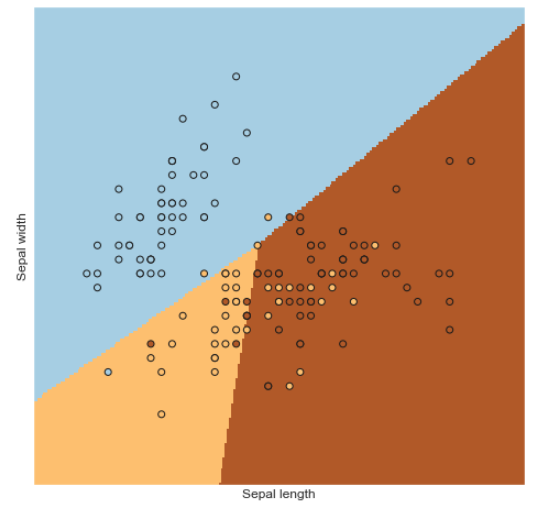至此多分类决策边界的绘制就介绍完毕。此处留有一个问题：如果不使用前两个字段进行绘制，而是选择其他的两个字段，输出结果如何呢？在进行特征字段选取的时候将列数进行改变即可，比如[0,2],[0,3],[1,2],[1,3],[2,3]还有5种情况没有进行测试

features3 = iris3.iloc[:, :2].values
features4 = iris4.iloc[:, [0,2]].values
features5 = iris5.iloc[:, [0,3]].values
features6 = iris6.iloc[:, [1,2]].values
features7 = iris7.iloc[:, [1,3]].values
features8 = iris8.iloc[:, [2,3]].values


可能有个疑问：这里为啥只选两个字段？就是因为这里的决策边界是二维的，只能通过两个字段才能绘制出来直线，如果三个字段就是下面的三维决策平面的绘制。同理下面三维决策平面的绘制也面临同样的情况，就是选哪三个字段进行呈现，4个字段选择3个一共有4种选择方式。

### 3.3.3 三维决策平面的绘制

仅做了解，全部代码如下。

import numpy as np
import matplotlib.pyplot as plt
from sklearn import datasets
from mpl_toolkits.mplot3d import Axes3D

X = iris.data[:, :3]  # we only take the first three features.
Y = iris.target

#make it binary classification problem
X = X[np.logical_or(Y==0,Y==1)]
Y = Y[np.logical_or(Y==0,Y==1)]

model = LogisticRegression()
clf = model.fit(X, Y)

# The equation of the separating plane is given by all x so that np.dot(svc.coef_, x) + b = 0.
# Solve for w3 (z)
z = lambda x,y: (-clf.intercept_-clf.coef_*x -clf.coef_*y) / clf.coef_

tmp = np.linspace(-5,5,30)
x,y = np.meshgrid(tmp,tmp)

fig = plt.figure()
ax.plot3D(X[Y==0,0], X[Y==0,1], X[Y==0,2],'ob')
ax.plot3D(X[Y==1,0], X[Y==1,1], X[Y==1,2],'sr')
ax.plot_surface(x, y, z(x,y))
ax.view_init(30, 60)
plt.show()


输出结果如下。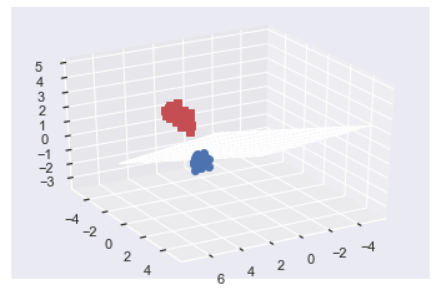最后就是这种二维或者三维的决策边界都是只选取了原特征数据中的部分字段，无论怎么进行模型的分类最后的模型得分始终超不过使用全部字段训练的模型的结果，也就是最开始的模型model.score()输出的结果：0.967。但是这种二维或者三维的决策边界的绘制是有意义的，后续的实战案例中的数据字段会有很多，但是并不是每一个字段都是对结果有着影响，因此就可以进行降维的方式将有效的信息进行提取，数据的维度降低后，就可以再进行决策边界的展示。

展开全文机器学习 逻辑回归 分类
• 而散点图更是包含着属于不同类别的数据点(用颜色或形状表示)，决策边界可以通过多种不同的策略绘制： 单线决策边界:在散点图上绘制决策边界的基本策略是找到一条将数据点分隔成不同类区域的单线。现在，利用训练过的...

作者——Navoneel Chakrabarty

在数据科学领域，分类问题是一个非常普遍和重要的问题。例如:糖尿病视网膜病变、情绪分析、数字识别、癌症类型预测(恶性或良性)等。这些问题往往通过机器学习或深度学习来解决。像糖尿病视网膜病变或青光眼检测这样的项目中，纹理分析经常被使用，而不是传统的图像处理或深度学习等。虽然根据研究论文，深度学习在处理糖尿病视网膜病变方面有着极强的优越性。

补充：纹理分析指通过一定的提取出纹理特征参数，从而获得纹理的定量或定性描述的处理过程·纹理分析方法按其性质而言，可分为两大类：统计分析方法和结构分析方法。纹理分析在遥感图像、 X、细胞和处理方面有广泛的应用。关于纹理，还没有一个统一的数学模型。它起源于表征纺织品表面性质的纹理概念，可以用来描述任何物质组成成分的排列情况，例如医学上X 射线照片中的、血管纹理、航天(或航空)地形照片中的岩性纹理等。图像处理中的视觉纹理通常理解为某种基本模式(色调基元)的重复排列。现在，我们再回到主题。相关研究论文如下：

"糖尿病视网膜病变检测的深度学习方法"链接：https://ieeexplore.ieee.org/document/8596839

在分类问题中，一个特定类的预测往往要涉及多个类。换句话说，它还可以以这样一种方式构建:将特定实例(特征空间几何中的数据点)保存在特定区域下(某一类)，并且与其他区域分离(其他类)。这种与其他区域的分离的"现象"我们称之为决策边界的可视化。在特征空间中决策边界的可视化是在散点图上完成的。其中每个点表示数据集的一个数据点，轴线表示特征。决策边界将数据点划分为多个区域，这些区域就是(我前面所谈及的)数据点所属的类。

决策边界的重要性/意义：

在使用数据集训练机器学习模型之后，我们通常需要可视化特征空间中数据点的类。散点图上的决策边界就是出于这个目的。而散点图更是包含着属于不同类别的数据点(用颜色或形状表示)，决策边界可以通过多种不同的策略绘制：

单线决策边界:在散点图上绘制决策边界的基本策略是找到一条将数据点分隔成不同类区域的单线。现在，利用训练过的模型找到与机器学习算法相关的参数，进而找到这条直线。然后利用得到的参数和机器学习算法找到直线坐标。如果你不知道ML算法的工作原理，那么你将无法继续进行下去。

基于轮廓的决策边界:另一种策略是绘制轮廓，这些轮廓是用匹配或紧密匹配的颜色包围数据点的区域——描绘数据点所属的类，以及描绘预测类的轮廓。这是最常用的策略，因为它不使用模型训练后得到的机器学习算法的参数和相关计算。但另一方面，我们并不能很好地用一条直线来分离数据点，也就是说这条直线只能通过训练后得到的参数及其坐标计算得到。

单线决策边界的范例演练：

在这里，我将演示基于逻辑回归的机器学习模型的单线决策边界。

进入逻辑回归假设其中z被定义为：theta_1、theta_2 theta_3,....其中，theta_n为逻辑回归的参数，x_1、x_2、…、x_n为特征。

因此，h(z)是一个Sigmoid函数，其范围是从0到1(包括0和1)。

在绘制决策边界时，取h(z) = Logistic回归中使用的阈值，通常为0.5。即：然后：现在，在绘制决策边界时，需要考虑两个特征，并沿散点图的x轴和y轴绘制。所以，也就是说其中x_1是数据集的原始特征

因此，得到2个x ' _1的值以及2个相应的x ' _2的值。x ' _1是单线决策边界的x极值，x ' _2是单线决策边界的y极值。

在虚构数据集上的应用：

该数据集包含100名学生在2次考试中获得的分数和标签(1，0)，该标签指示该学生是否将被大学录取(用1和0表示)。相关数据集链接：https://github.com/navoneel1092283/logistic_regression.git

问题陈述:"根据在两次考试中获得的分数，用逻辑回归预测该学生是否会被大学录取。"

在这里，两门考试的分数将是考虑的两个特征。

下面是具体的实现方法：

相关链接：https://hackernoon.com/logistic-regression-in-python-from-scratch-954c0196d258对数据集进行逻辑回归:得到(参数)向量，获取数据点的预测或预测类：绘制单线决策边界：得到单线决策边界

这样，任何基于逻辑回归的机器学习模型都可以绘制出单线决策边界。对于基于其他机器学习算法的模型而言，则必须知道相应的假设等等。

基于轮廓的决策边界的范例演练：

利用刚才的数据集和训练模型，绘制基于轮廓的决策边界。得到基于轮廓的决策边界，其中黄色->允许，蓝色->不允许

这种方法显然更方便，因为机器学习算法不需要假设，也不需要数学。所需要的只是高级Python编程的诀窍。

因此，它是绘制任意机器学习模型决策边界的一种通用方法。

在现实生活中和一些高级项目中，都会涉及到许多特征。那么，如何在二维散点图中绘制决策边界呢?

面对这种情况，我认为有多种解决办法：

1.利用随机森林分类器等给特征进行重要性评分，得到2个最重要的特征，然后在散点图上绘制决策边界。

2.主成分分析(PCA)或线性判别分析(LDA)等降维技术可用于将N个特征嵌入到2个特征中，从而将N个特征的信息解释或减少为2个特征(n_components = 2)。然后再基于这两个特征在散点图上绘制决策边界。

这就是决策边界可视化。

展开全文• 定义一个边界决策函数 1 #咱们先顶一个一个函数来画决策边界 2 defplot_decision_boundary(pred_func):3 4 #设定最大最小值，附加一点点边缘填充 5 x_min, x_max = X[:, 0].min() - .5, X[:, 0].max() + .5 6 y_min...
• 凌云时刻 · 技术导读：决策边界顾名思义就是需要分类的数据中，区分不同类别的边界，举个不恰当的例子，就像省的地界一样，你处在北京还是处在河北，全看你站在区分北京和河北的那条线的哪边。这节...
• 0x00 前言 在逻辑回归算法中，可以求出各个参数的系数和截距，即参数组值。那这个参数有几何意义么？在本篇文章中会引出分类文中非常重要的一个概念：决策边界。通过对决策边界的学习，可以更加...
• SVM决策边界 一、线性可分 w = clf.coef_ #调用coef_取得w值 a = -w / w xx = np.linspace(-2, 2) # x轴范围 yy = a * xx - (clf.intercept_ / w) b = clf.support_vectors_ yy_down = a * xx + ...机器学习 python
• clf.fit(xtrain,ytrain) from mlxtend.plotting import plot_decision_regions plt.figure() fig = plot_decision_regions(X = xtrain,y = ytrain,clf = clf) plt.show()算法 python
• 1⃣️决策边界 2⃣️拟合参数 3⃣️实现算法 1⃣️ ⚠️决策边界是假设函数的属性不是数据集的属性 ❓如何得到决策边界 答：只要有确定的theta 就可确定决策边界 确定边界的整个过程 通过训练集对数据进行...机器学习
• 决策树1. KNN算法流程2. KNN改进约会网站的配对效果2.1 数据准备：从文本中解析数据2.2 数据可视化：散点图2.3 数据处理：归一化数值2.4 构建KNN模型对测试集前10个样本进行预测寻找测试样本的K-近邻2.5 模型使用：...机器学习
• 目录1、分类问题2、逻辑回归3、损失函数4、决策边界4、OvR与OvO 1、分类问题 机器学习中有三大问题，分别是回归、分类和聚类。线性回归属于回归任务，而逻辑回归和k近邻算法属于分类任务。逻辑回归算法主要应用于...机器学习
• 为了得到离散的0或1分类，我们可以将假设函数的输出翻译如下： 我们的逻辑函数g的行为方式是，当它的输入大于或等于零时...决策边界是分隔y＝0和y＝1的区域的直线。它是由我们的假设函数创建的。例子: ...
• 决策边界（Decision boundary） 逻辑回归虽然带着回归两字却和线性回归有很大的区别，线性回归主要用于预测问题，其输出值为连续变量，而logistic回归主要用于分类问题，其输出值为离散值。logistic回归可以用于...逻辑回归 目标函数 凸函数 梯度下降
• 决策边界   上一篇博客我们实现了自己的逻辑回归算法，将鸢尾花这个数据集进行了很好的分类识别。当然，我们只选取了其中的两个类和两个特征。   对于 SigmoidSigmoidSigmoid 这个式子：      通过观察 ...
• 最近在做有关逻辑回归的作业，需要绘制决策边界。绘制原理是： 对于逻辑回归，其决策边界为θTX=0\theta^TX = 0θTX=0处，其中θ=[θ0,θ1,θ2,⋯ ,θn];X=[X0,X1,X2,⋯ ,Xn]\theta = [\theta_0,\theta_1,\theta_2...python
• 如果我们将多个核函数组合在一起，就可以构造出一个复杂的非线性决策边界。那么，如何选取核函数的核呢？ 一个简单的做法就是，把所有样本点都作为核，一个样本的特征值就是它与所有样本点的相似度。具体来讲，我们...支持向量机 机器学习 人工智能
• 作者主页(文火冰糖的硅基工坊)：文火冰糖（王文兵）的博客_...3.4 生成每个输入的样本点到决策边界的距离 第4步骤：通过等高线可视化决策边界与隔离带 4.1 什么是网格 4.2 堆叠 4.3 绘制原始样本和决策边界 4.4 绘机器学习 支持向量机
• 前言：本文主要为简单介绍决策边界，以及使用自定义函数绘制决策边界，不涉及决策边际的深度探讨。 内容分为两个模块：1. 介绍什么是决策边界；2. 如何通过自定义函数绘制逻辑回归的决策边界。 如何理解决策边界...逻辑回归 机器学习 python
• 损失函数： 真实为 1 就应该 sigmoid 尽量大 真实为 0 就应该1- sigmoid 尽量大 此种情况下损失函数尽可能小 绘制损失函数图像：编程实现： #绘制...决策边界可以在一定程度帮我们查看模型是否过于复杂了，过拟合问题逻辑回归 机器学习 python
• 决策边界：在特征空间内，根据不同特征对样本进行分类，不同类型间的分界就是模型针对该数据集的决策边界决策边界，用于分类问题中，通过决策边界可以更好的可视化分类结果； 在二维特征空间中，决策......